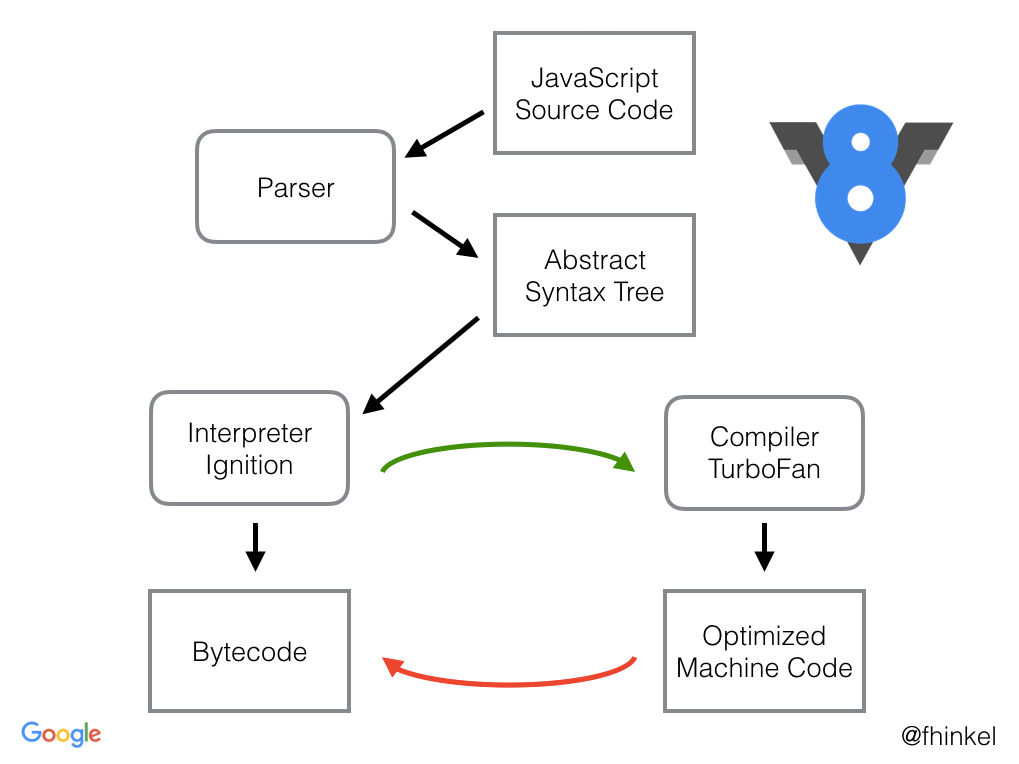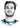# Bugs in V8's Exponentiation Operator

Categories:

I always thought that the new ES6 exponentiation operator `x ** y` is the same as `Math.pow(x,y)`.

Indeed this is what the specification says about `Math.pow`:

Return the result of Applying the ** operator with base and exponent as specified in 12.6.4.

12.6.4 - Applying the ** Operator states that the result is implementation-dependent - but there should still be no discrepancy between `**` and `Math.pow`.

However, evaluating the following in the current V8 JS Engine (Chrome / Node) results in this:

``````console.log('1.35 ** 92', 1.35 ** 92)                   // 978828715394.7672
console.log('Math.pow(1.35, 92)', Math.pow(1.35, 92))   // 978828715394.767``````

The exponentiation operator `**` returns a more accurate approximation.

But this is not the only weirdness with the exponentiation operator: Let’s try evaluating the same with variables (REPL) - it shouldn’t make any difference:

``````const exponent = 92;
console.log(`1.35 ** exponent`, 1.35 ** exponent)                   // 978828715394.767
console.log('1.35 ** 92', 1.35 ** 92)                               // 978828715394.7672
console.log(`Math.pow(1.35, exponent)`, Math.pow(1.35, exponent))   // 978828715394.767
console.log('Math.pow(1.35, 92)', Math.pow(1.35, 92))               // 978828715394.767``````

But it does: `1.35 ** 92` differs from `1.35 ** exponent`.

So what seems to be happening here, is that the JS code `1.35 ** 92` is processed by the compiler and already constant folded.

This makes sense as V8 really compiles to machine code.

V8 increases performance by compiling JavaScript to native machine code before executing it, versus executing bytecode or interpreting it.

V8 works by first interpreting the JS code with their Ignition Interpreter and having a second run with the TurboFan compiler optimizing the machine code.TurboFan now does constant folding and its exponentiation algorithm has a better precision than the JIT compiler’s (Ignition) exponentiation algorithm.

If you try the same in other JS engines like Firefox’s SpiderMonkey, the result is a consistent value of `978828715394.767` among all computations.

## Is it a bug?

I would say so, although it wasn’t severe in my code, it’s still not following the spec that says `Math.pow` and `**` should result in the same value.

If you’re transpiling the code with babel, `x ** y` is translated to `Math.pow(x,y)` which again leads to discrepancies between transpiled and untranspiled code. As we have seen `Math.pow(1.35, 92)` is not being optimized - (only operators seem to be optimized by V8), and thus `1.35 ** 92` results in different code when transpiled to ES5.

Using this bug and disregarding any clean code practices, we can write a nice function to determine if we’re running on Chrome (unless you transpile your code 😉):

``````function isChrome() {
return 1.35 ** 92 !== Math.pow(1.35, 92)
}``````

Still more readable than user agent strings. 🤷

# Hi, I'm Christoph Michel 👋I'm a , , and .

I mostly do and help small to mid-sized teams from all over the world 🌎 on both short and long-term projects on an independent contractor basis.

I strive for efficiency ⏱️ and therefore track many aspects of my life.